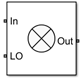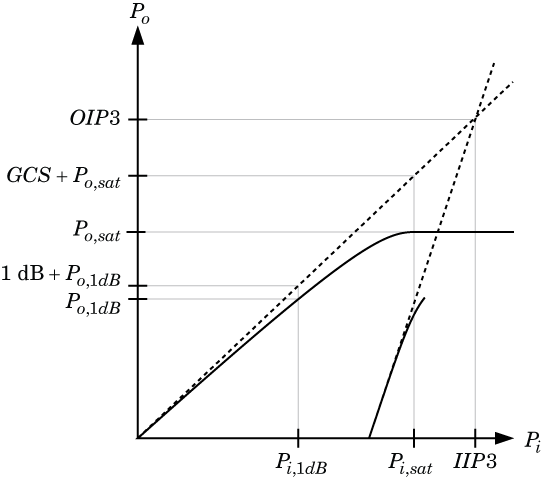# Mixer

Model mixer in RF systems

• Library:
• RF Blockset / Circuit Envelope / Elements

•## Description

The Mixer block performs signal frequency translation and nonlinear amplification.

For a given RF input signal, VRF = ARFcos(ωRFt) and an LO input signal VLO = AcLOcos(ωLOt), the mixer multiplies the signals at the input ports:

`$\begin{array}{c}{V}_{\text{in}}{V}_{\text{LO}}={A}_{\text{in}}\mathrm{cos}\left({\omega }_{\text{in}}t\right){A}_{\text{LO}}\mathrm{cos}\left({\omega }_{\text{LO}}t\right)\\ =\frac{{A}_{\text{in}}{A}_{\text{LO}}}{2}\mathrm{cos}\left[\left({\omega }_{\text{in}}+{\omega }_{\text{LO}}\right)t\right]+\frac{{A}_{\text{in}}{A}_{\text{LO}}}{2}\mathrm{cos}\left[\left({\omega }_{\text{in}}-{\omega }_{\text{LO}}\right)t\right]\end{array}$`

This mixing converts the frequency of RF signal to ωRF + ωLO and ωRF – ωLO. For the mixer to perform this operation correctly, you must include the frequencies ωRF + ωLO or ωRF – ωLO in the simulation frequencies the Configuration block calculates.

The Power gain specification for this block relates the power of a single-sideband (SSB) to the input.

After mixing the RF and LO signals, the mixer block performs amplification. To model linear amplification, the mixer scales the signals by the coefficient a1. A Voltage Controlled Voltage Source (VCVS), specified with a polynomial, implements nonlinear amplification. The polynomial includes saturation automatically and produces additional intermodulation frequencies.

Mixer block mask icons are dynamic and indicate the current state of the applied noise parameter. This table shows you how the icons on this block vary based on the state of the Noise figure (dB) parameter on the block.

Noise figure (dB): `10`Noise figure (dB): `0`## Parameters

expand all

Main

Source parameter of conversion gain, specified as one of the following:

• `Available power gain` — The block uses the value of the Available power gain parameter to calculate the linear voltage gain term of the polynomial VCVS, a1. This calculation assumes a matched load termination for the mixer.

• `Open circuit voltage gain` — The block uses the value of the Open circuit voltage gain parameter as the linear voltage gain term of the polynomial VCVS, a1.

• `Polynomial coefficients` — The block implements a nonlinear voltage gain according to the polynomial you specify. The order of the polynomial must be less than or equal to 9 and the coefficients are ordered in ascending powers. If a vector a has 10 coefficients, [a0, a1, a2, …, a9], the polynomial it represents is Vout = a0 + a1 Vin + a2 Vin2+ ⋯ + a9 Vin9. In this case, a1 represents the linear gain term, and the modeling of higher-order terms is done according to .

For example, the vector [a0, a1, a2, a3] specifies the relation Vout = a0 + a1 Vin + a2 Vin2 + ⋯ + a3 Vin3.

Trailing zeroes are omitted: if a3 = 0, [a0, a1, a2] defines the same polynomial as [a0, a1, a2, 0]. The default value of this parameter is [0 1], corresponding to the linear relation Vo = Vi.

Linear gain of mixer, specified as a scalar in dB. Specify the units from the corresponding drop-down list.

#### Dependencies

To enable this parameter, select ```Available power gain``` in Source of conversion gain tab.

Open circuit voltage of mixer, specified as a scalar in dB. Specify the units from the corresponding drop-down list.

#### Dependencies

To enable this parameter, select ```Open circuit voltage gain``` in Source of conversion gain tab.

Order of polynomial, specified as a vector.

The order of the polynomial must be less than or equal to 9. The coefficients are ordered in ascending powers. If a vector has 10 coefficients, ```[a0,a1,a2, ... a9]```, the polynomial it represents is:

Vout = a0 + a1Vin + a2Vin2 + ...  + a9Vin9

where a1 represents the linear gain term, and higher-order terms are modeled according to .

For example, the vector `[a0,a1,a2,a32]` specifies the relation Vo = a0 + a1V1 + a2V12 + a3V13. Trailing zeroes are omitted. If a3 = 0, then `[a0,a1,a2]` defines the same polynomial as [a0,a1,a2,0]. The default value of this parameter is [0,1], corresponding to the linear relation Vo = Vi.

#### Dependencies

To enable this parameter, select `Polynomial coefficients` in Source of conversion gain tab.

Input impedance of mixer, specified as a scalar.

Output impedance of mixer, specified as a scalar.

Output impedance of mixer, specified as a scalar.

Single-sideband noise figure of mixer, specified as a scalar according to the IEEE® definition.

To model noise in circuit envelope model with a Noise, Amplifier, or Mixer block, you must select the Simulate noise check box in the Configuration block dialog box.

The IEEE SSB definition assumes that the noise in the image bandwidth at the input of the mixer is perfectly rejected, while the mixer internally generates noise in both the image bandwidth and the signal bandwidth. As a result, the noise at the output of the mixer is the sum of two contributions:

`${N}_{\text{out}}={N}_{\text{in}}{G}_{\text{mix}}+2{N}_{\text{mixer}}{G}_{\text{mix}},$`

where:

• Nout is the noise at the output of the mixer.

• Nin is the noise at the input of the mixer (assuming that the noise in the image bandwidth is perfectly rejected).

• Nmixer is the noise internally generated by the mixer in both the signal and the image bandwidths.

• Gmix is the mixer gain.

As a result, the noise factor according to the IEEE SSB definition can be expressed as

`${F}_{\text{SSB-IEEE}}=1+2{N}_{\text{mixer}}/{N}_{\text{in}},$`

which is related to other commonly used definitions through

`$\begin{array}{c}{F}_{\text{SSB}}=2+2{N}_{\text{mixer}}/{N}_{\text{in}}=1+{F}_{\text{SSB-IEEE}},\\ {F}_{\text{DSB}}=1+{N}_{\text{mixer}}/{N}_{\text{in}}=\frac{1}{2}\left(1+{F}_{\text{SSB-IEEE}}\right).\end{array}$`

You can apply the IEEE SSB definition directly to describe mixer stages when using the Friis formulas for link budget analysis. Using the other definitions requires changing the Friis formulas. Both the Mixer block in RF Blockset™ and the RF Budget Analyzer app in RF Toolbox™ use the IEEE definition.

The analytic relationships between the three definitions allow you to simulate the noise level at the output of the mixer. For example,

If you simulate an RF Blockset mixer without including an ideal image rejection filter, then the noise at the output of the mixer is larger than that predicted by the noise figure, because the noise in the image bandwidth is effectively folded onto the output signal.

For this reason, when generating models, both the Modulator and Demodulator blocks insert an ideal image-rejection filter automatically. (You can remove the filtering within the block mask.)

The Noise Figure Testbench block measures the SSB noise figure and enables you to verify that the simulated noise figure has the expected value.

• If you add an ideal image rejection filter to your model, then the effective noise figure is consistent with the analytic value.

• If you remove the image-rejection filter, or if you use a filter with partial rejection, then the measured noise figure is larger than the analytic value.

Select this parameter to internally ground and hide the negative terminals. To expose the negative terminals, clear this parameter. By exposing these terminals, you can connect them to other parts of your model.

By default, this option is selected.

Nonlinearity

Polynomial nonlinearity, specified as one of the following:

• `Even and odd order`: When you select `Even and odd order`, the amplifier can produce second- and third-order intermodulation frequencies in addition to a linear term.

• `Odd order`: When you select ```Odd order```, the amplifier generates only odd order intermodulation frequencies.

The linear gain determines the linear a1 term. The block calculates the remaining terms from the specified parameters. These parameters are IP3, 1-dB gain compression power, Output saturation power, and Gain compression at saturation. The number of constraints you specify determines the order of the model. The figure shows the graphical definition of the nonlinear mixer parameters.Intercept points convention, specified as `Input`-referred or `Output`-referred. Use this specification for the intercept points, 1-dB gain compression power, and saturation power.

Second-order intercept point. specified as a scalar. The default value `inf` `dBm` corresponds to an unspecified point.

#### Dependencies

To enable this parameter, select `Even and odd order` in Nonlinear polynomial type tab.

Third-order intercept point, specified as a scalar. The default value `inf` `dBm` corresponds to an unspecified point.

1-dB gain compression power, specified as a scalar. The 1-dB gain compression point must be less than the output saturation power.

#### Dependencies

To enable this parameter, select `Odd order` in Nonlinear polynomial type tab.

Output saturation power, specified as a scalar. The block uses this value to calculate the voltage saturation point used in the nonlinear model. In this case, the first derivative of the polynomial is zero, and the second derivative is negative.

#### Dependencies

To enable this parameter, select `Odd order` in Nonlinear polynomial type tab.

Gain compression at saturation, specified as a scalar.

#### Dependencies

To enable this parameter, select `Odd order` in Nonlinear polynomial type tab and set Output saturation power .

 Grob, Siegfried, and Jürgen Lindner. “Polynomial Model Derivation of Nonlinear Amplifiers.” Department of Information Technology, University of Ulm, Germany.